# Trigonometric Ratios of Standard Angles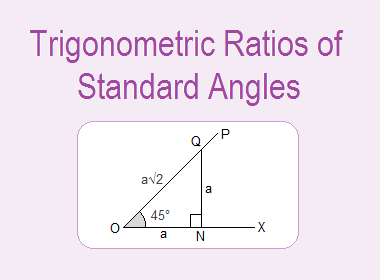## Trigonometric Ratios of Standard Angles

In trigonometry, 0°, 30°, 45°, 60°, 90°, 120°, 135°, 150°, 180° etc. are considered as the standard angles. We can derive the values of trigonometric ratios of standard angles geometrically.

********************

10 Math Problems officially announces the release of Quick Math Solver, an Android App on the Google Play Store for students around the world.

********************

We should remember those values for the calculations in trigonometry. Here is the geometrical procedure for the derivation of trigonometric ratios of standard angles.

### Trigonometric ratios of 45°

Let OX be the initial line and trace out an XOP = 45°. From Q, a point on OP, draw QNOX. Then NQO = 90° – 45° = 45°. Therefore it is an isosceles triangle, i.e. ON = NQ. Let ON = NQ = a.From the right angled triangle ONQ, using Pythagoras relation,

OQ2 = ON2 + NQ2

= a2 + a2

= 2a2

OQ  = a√2

Now for right angled triangle ONQ with angle of reference NOQ = 45°,### Trigonometric ratios of 60° and 30°

Let ABC be a triangle in which AB = BC = CA and hence A = B = C = 60°. From A draw ADBC. Then BAD = CAD = 30° and BD = CD. Let AB = BC = CA = 2a. So, BD = CD = a.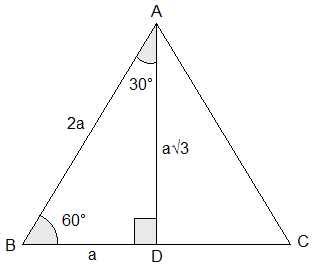Now, from right angled DABD,

= 4a2 – a2

= 3a2

Now, in right angled DABD with angle of reference ABD = 60°,And, with the angle of reference BAD = 30°,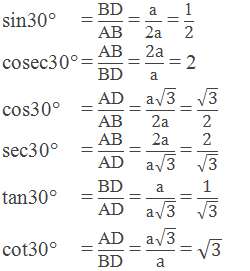### Trigonometric ratios of 0°

Let OX be the initial line and trace out an XOP = θ. Let Q be a point on OP. Draw QMOX.When θ is decreased and tends to be 0° keeping OM constant, then QM = 0 and OQ = OM.

Now, from right angled triangle MOQ with angle of reference θ = 0°,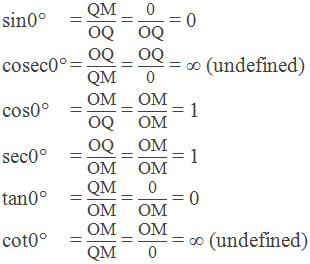### Trigonometric ratios of 90°

Let OX be the initial line and trace out an XOP = θ. Let Q be a point on OP. Draw QMOX.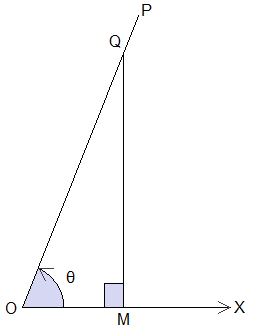When θ is increased and tends to be 90° keeping MQ constant, then OM = 0 and OQ = MQ.

Now, from right angled triangle MOQ with angle of reference θ = 90°,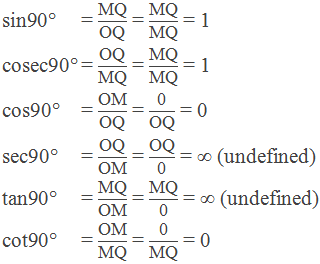### Table of Trigonometric Ratios of Standard Angles### Worked Out Examples

Example 1: Find the value of sin230° + sin245° + sin260°

Solution: Here,Example 2: Find the value of:Solution: Here,Example 3: Prove that:Solution: Here,Example 4: Find the value of x: sin60°cos30° + x sin45°cos45° = sin30°cos60°

Solution: Here,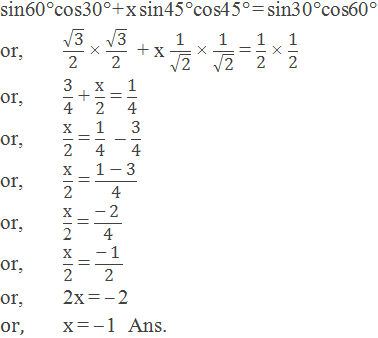Example 5: In the given figure, the distance between house B and House C is 20m and BCA = 30°. Calculate the distance between house A and B.

Solution: Here,

ΔABC is a right angled triangle. BC = 20m, BCA = 30° and BAC = 90°Sin30° = AB/BC

or,     ½ = AB/20m

or,     2AB = 20m

or,     AB = 20m/2

or,     AB = 10m

Hence, the distance between house A and B is 10m.

If you have any question or problems regarding the Trigonometric Ratios of Standard Angles, you can ask here, in the comment section below.